# RD Sharma Solutions for Class 6 Chapter 7: Decimals Exercise 7.9

The students get an overall idea about the concepts which are covered in this chapter by using RD Sharma Solutions Class 6. Exercise 7.9 has problems based on subtraction of numbers with decimals along with illustrative examples which help students improve their problem-solving abilities. The students understand the important concepts based on CBSE exam pattern using RD Sharma Solutions for Class 6 Maths Chapter 7 Decimals Exercise 7.9 PDF which are given below.

## RD Sharma Solutions for Class 6 Chapter 7: Decimals Exercise 7.9 Download PDF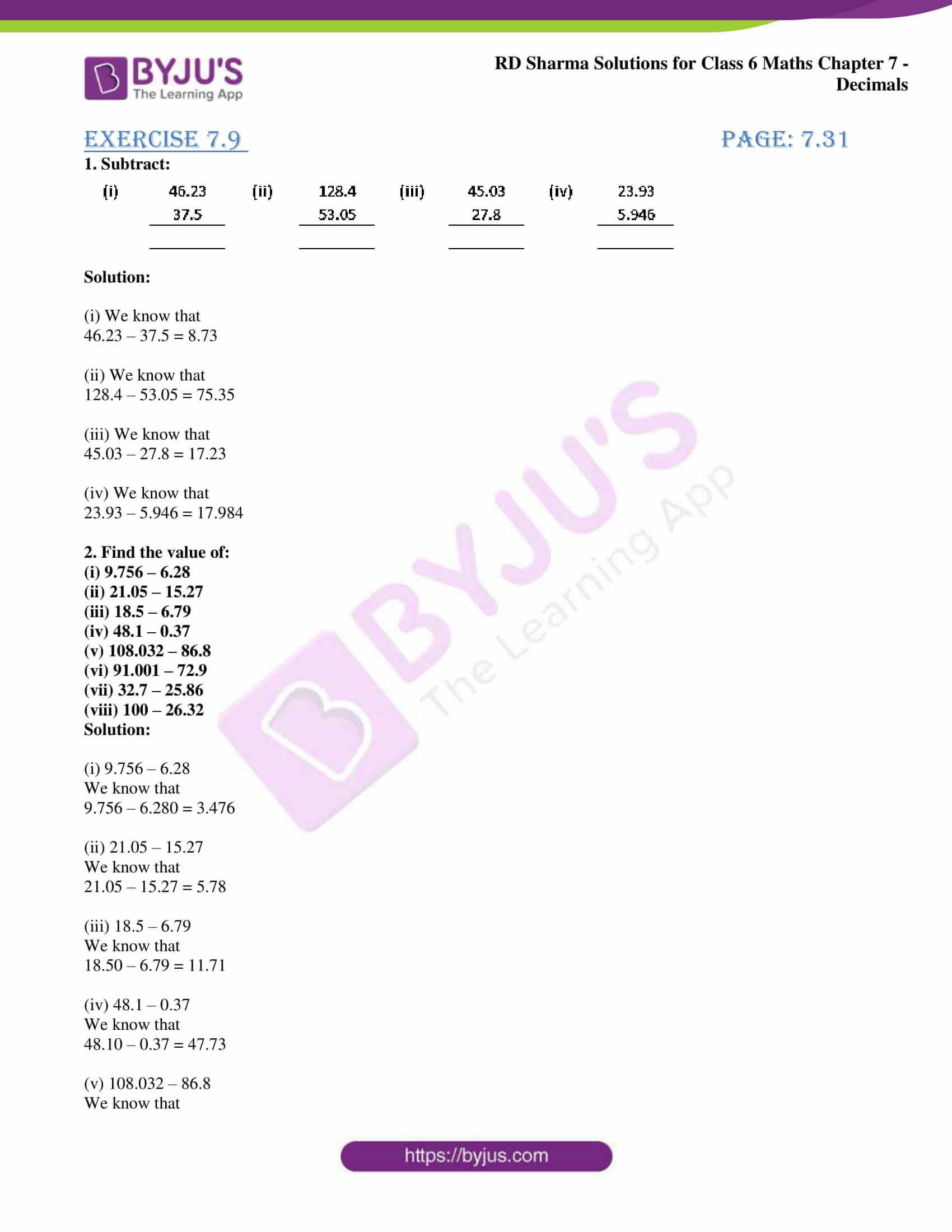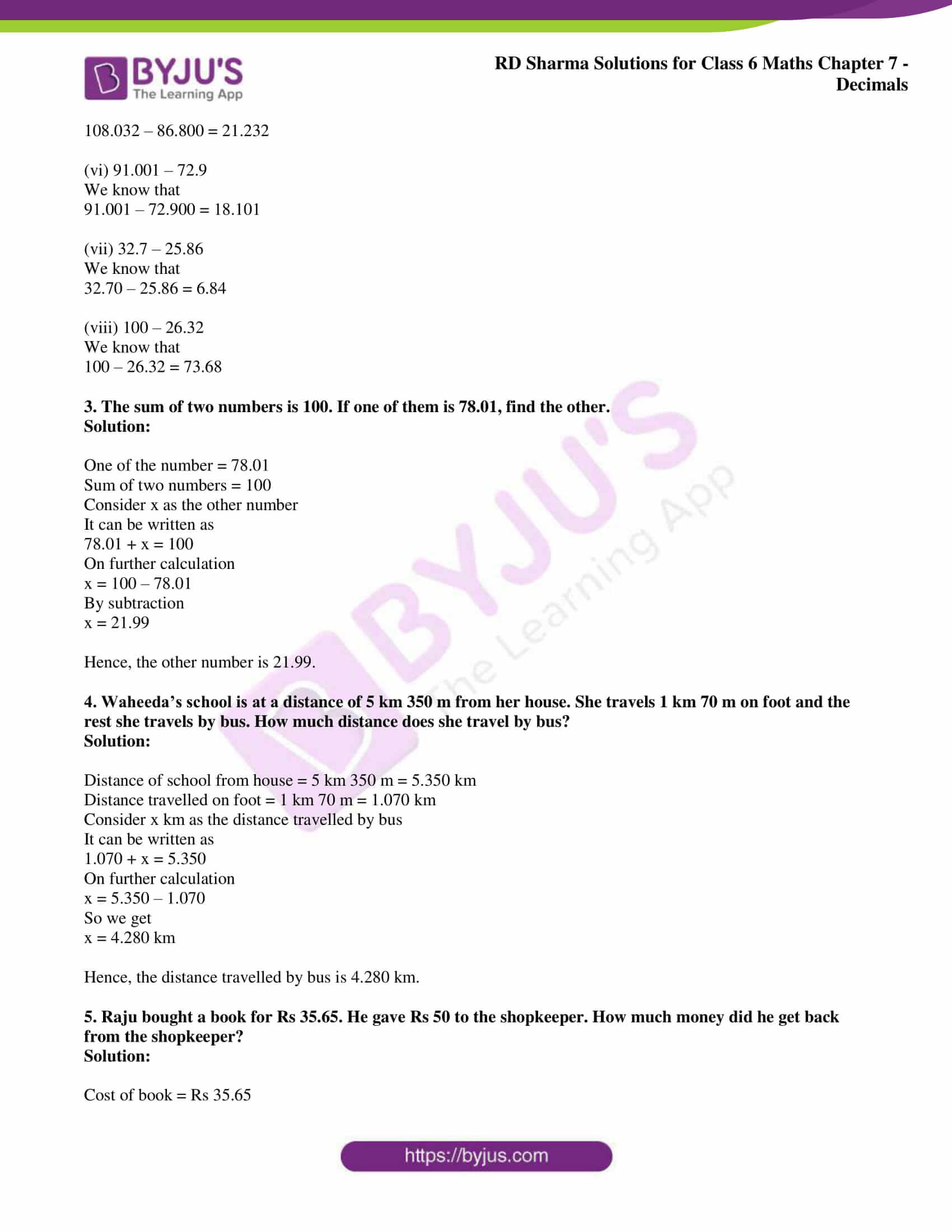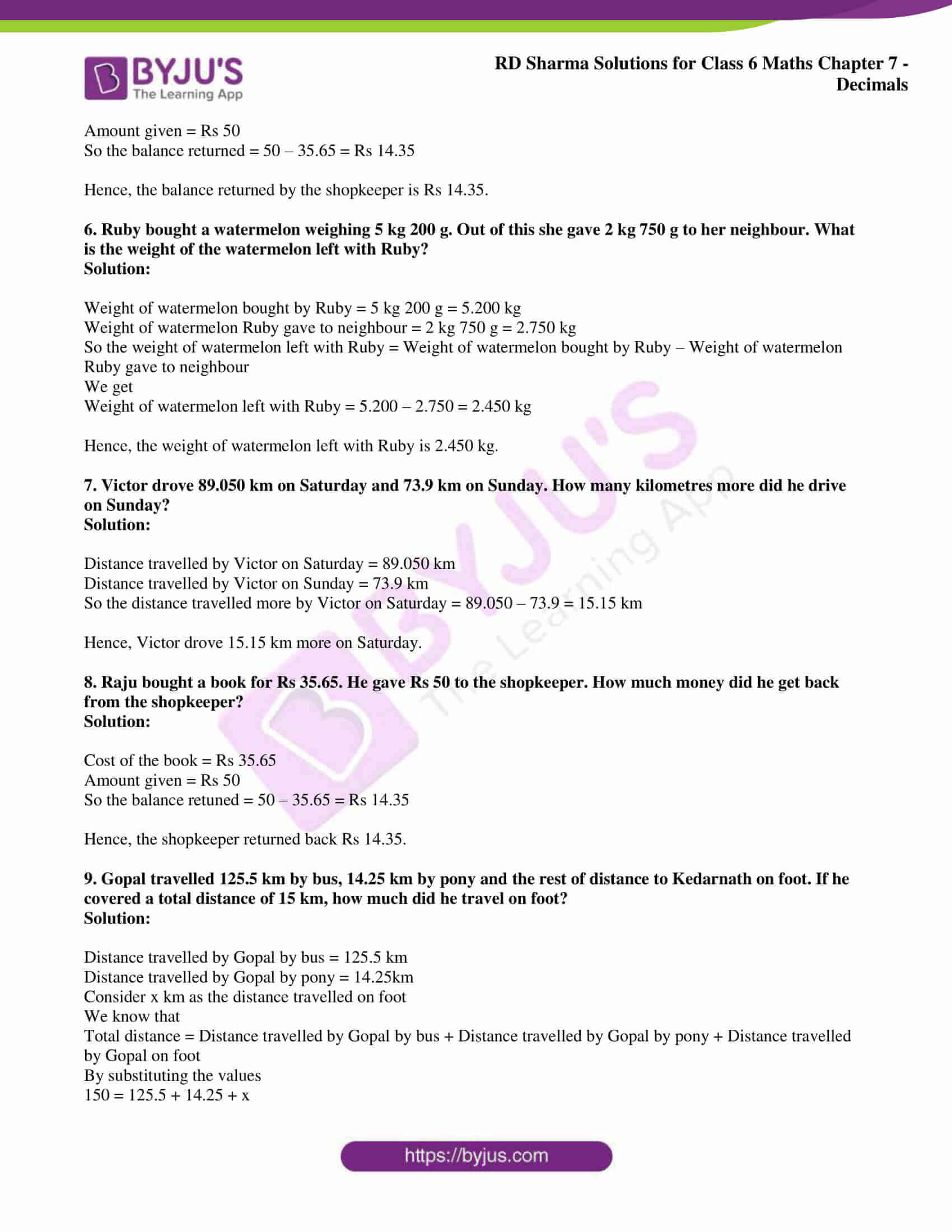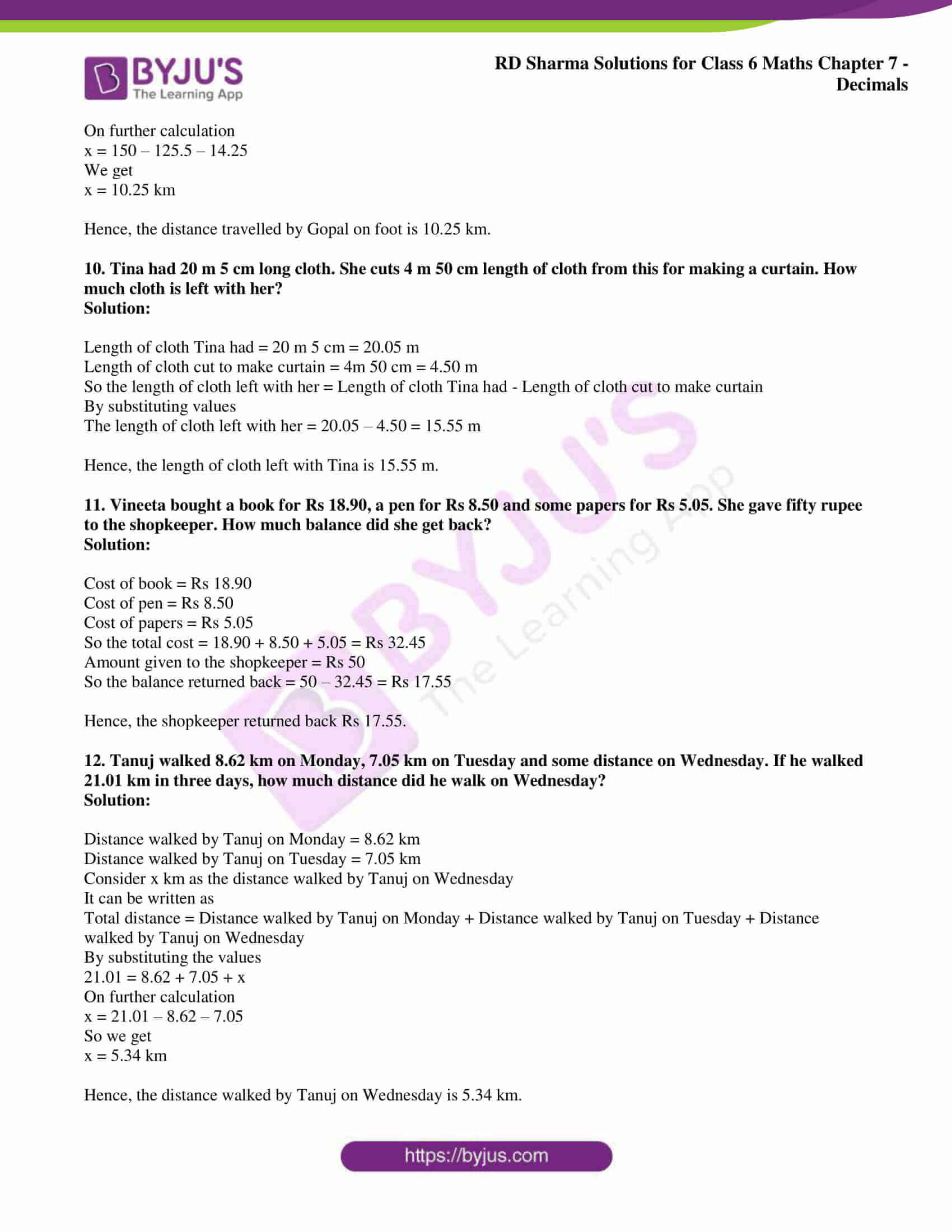### Access RD Sharma Solutions for Class 6 Chapter 7: Decimals Exercise 7.9

1. Subtract: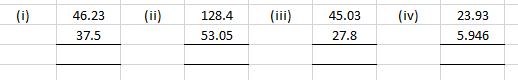Solution:

(i) We know that

46.23 – 37.5 = 8.73

(ii) We know that

128.4 – 53.05 = 75.35

(iii) We know that

45.03 – 27.8 = 17.23

(iv) We know that

23.93 – 5.946 = 17.984

2. Find the value of:

(i) 9.756 – 6.28

(ii) 21.05 – 15.27

(iii) 18.5 – 6.79

(iv) 48.1 – 0.37

(v) 108.032 – 86.8

(vi) 91.001 – 72.9

(vii) 32.7 – 25.86

(viii) 100 – 26.32

Solution:

(i) 9.756 – 6.28

We know that

9.756 – 6.280 = 3.476

(ii) 21.05 – 15.27

We know that

21.05 – 15.27 = 5.78

(iii) 18.5 – 6.79

We know that

18.50 – 6.79 = 11.71

(iv) 48.1 – 0.37

We know that

48.10 – 0.37 = 47.73

(v) 108.032 – 86.8

We know that

108.032 – 86.800 = 21.232

(vi) 91.001 – 72.9

We know that

91.001 – 72.900 = 18.101

(vii) 32.7 – 25.86

We know that

32.70 – 25.86 = 6.84

(viii) 100 – 26.32

We know that

100 – 26.32 = 73.68

3. The sum of two numbers is 100. If one of them is 78.01, find the other.

Solution:

One of the number = 78.01

Sum of two numbers = 100

Consider x as the other number

It can be written as

78.01 + x = 100

On further calculation

x = 100 – 78.01

By subtraction

x = 21.99

Hence, the other number is 21.99.

4. Waheeda’s school is at a distance of 5 km 350 m from her house. She travels 1 km 70 m on foot and the rest she travels by bus. How much distance does she travel by bus?

Solution:

Distance of school from house = 5 km 350 m = 5.350 km

Distance travelled on foot = 1 km 70 m = 1.070 km

Consider x km as the distance travelled by bus

It can be written as

1.070 + x = 5.350

On further calculation

x = 5.350 – 1.070

So we get

x = 4.280 km

Hence, the distance travelled by bus is 4.280 km.

5. Raju bought a book for Rs 35.65. He gave Rs 50 to the shopkeeper. How much money did he get back from the shopkeeper?

Solution:

Cost of book = Rs 35.65

Amount given = Rs 50

So the balance returned = 50 – 35.65 = Rs 14.35

Hence, the balance returned by the shopkeeper is Rs 14.35.

6. Ruby bought a watermelon weighing 5 kg 200 g. Out of this she gave 2 kg 750 g to her neighbour. What is the weight of the watermelon left with Ruby?

Solution:

Weight of watermelon bought by Ruby = 5 kg 200 g = 5.200 kg

Weight of watermelon Ruby gave to neighbour = 2 kg 750 g = 2.750 kg

So the weight of watermelon left with Ruby = Weight of watermelon bought by Ruby – Weight of watermelon Ruby gave to neighbour

We get

Weight of watermelon left with Ruby = 5.200 – 2.750 = 2.450 kg

Hence, the weight of watermelon left with Ruby is 2.450 kg.

7. Victor drove 89.050 km on Saturday and 73.9 km on Sunday. How many kilometres more did he drive on Sunday?

Solution:

Distance travelled by Victor on Saturday = 89.050 km

Distance travelled by Victor on Sunday = 73.9 km

So the distance travelled more by Victor on Saturday = 89.050 – 73.9 = 15.15 km

Hence, Victor drove 15.15 km more on Saturday.

8. Raju bought a book for Rs 35.65. He gave Rs 50 to the shopkeeper. How much money did he get back from the shopkeeper?

Solution:

Cost of the book = Rs 35.65

Amount given = Rs 50

So the balance retuned = 50 – 35.65 = Rs 14.35

Hence, the shopkeeper returned back Rs 14.35.

9. Gopal travelled 125.5 km by bus, 14.25 km by pony and the rest of distance to Kedarnath on foot. If he covered a total distance of 15 km, how much did he travel on foot?

Solution:

Distance travelled by Gopal by bus = 125.5 km

Distance travelled by Gopal by pony = 14.25km

Consider x km as the distance travelled on foot

We know that

Total distance = Distance travelled by Gopal by bus + Distance travelled by Gopal by pony + Distance travelled by Gopal on foot

By substituting the values

150 = 125.5 + 14.25 + x

On further calculation

x = 150 – 125.5 – 14.25

We get

x = 10.25 km

Hence, the distance travelled by Gopal on foot is 10.25 km.

10. Tina had 20 m 5 cm long cloth. She cuts 4 m 50 cm length of cloth from this for making a curtain. How much cloth is left with her?

Solution:

Length of cloth Tina had = 20 m 5 cm = 20.05 m

Length of cloth cut to make curtain = 4m 50 cm = 4.50 m

So the length of cloth left with her = Length of cloth Tina had – Length of cloth cut to make curtain

By substituting values

The length of cloth left with her = 20.05 – 4.50 = 15.55 m

Hence, the length of cloth left with Tina is 15.55 m.

11. Vineeta bought a book for Rs 18.90, a pen for Rs 8.50 and some papers for Rs 5.05. She gave fifty rupee to the shopkeeper. How much balance did she get back?

Solution:

Cost of book = Rs 18.90

Cost of pen = Rs 8.50

Cost of papers = Rs 5.05

So the total cost = 18.90 + 8.50 + 5.05 = Rs 32.45

Amount given to the shopkeeper = Rs 50

So the balance returned back = 50 – 32.45 = Rs 17.55

Hence, the shopkeeper returned back Rs 17.55.

12. Tanuj walked 8.62 km on Monday, 7.05 km on Tuesday and some distance on Wednesday. If he walked 21.01 km in three days, how much distance did he walk on Wednesday?

Solution:

Distance walked by Tanuj on Monday = 8.62 km

Distance walked by Tanuj on Tuesday = 7.05 km

Consider x km as the distance walked by Tanuj on Wednesday

It can be written as

Total distance = Distance walked by Tanuj on Monday + Distance walked by Tanuj on Tuesday + Distance walked by Tanuj on Wednesday

By substituting the values

21.01 = 8.62 + 7.05 + x

On further calculation

x = 21.01 – 8.62 – 7.05

So we get

x = 5.34 km

Hence, the distance walked by Tanuj on Wednesday is 5.34 km.